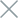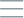• Home
• Tags
• Series
•# Optimizing and Organizing Tests with Mocha and Chai

In the software development process, optimizing and organizing tests is crucial to ensure accuracy and efficiency in the testing phase. In this article, we will explore how to optimize and organize tests with Mocha and Chai in Node.js.

Optimizing and organizing tests improves the testing process, reduces errors, and enhances the reliability of your application. By implementing these techniques, you can effectively manage and execute tests in your Node.js project using Mocha and Chai.

## Test Organization:

• Categorizing tests by functionality: Organizing tests based on functionality makes it easier to manage and identify the testing goals for each specific feature in your project.
• Utilizing nested describes: Utilize nested describes to create a hierarchical structure for organizing tests. This helps maintain a clear and readable structure for your test suite.

## Using hooks to perform setup and teardown tasks before and after tests

• Utilizing hooks: Mocha provides hooks such as `before`, `after`, `beforeEach`, and `afterEach` to perform pre and post-test operations. Using hooks helps save time and improve the overall performance of the tests.
• Using `skip` and `only` directives: The `skip` directive allows you to skip unnecessary tests during development. The `only` directive enables running specific tests, which is useful when you only need to test a small portion of the codebase.

Example:

``````describe('Calculator', () => {
beforeEach(() => {
// Set up data for all tests within this describe block
});

afterEach(() => {
// Clean up after running all tests within this describe block
});

it('should return the correct sum', () => {
});

it('should handle negative numbers', () => {
// Test addition with negative numbers
});
});

describe('Subtraction', () => {
it('should return the correct difference', () => {
// Test subtraction operation
});

it('should handle subtracting a larger number from a smaller number', () => {
// Test subtraction when subtracting a larger number from a smaller number
});
});
});
``````

## Grouping tests and using describe blocks for organization

To organize and group tests together, we can use the `describe` blocks in a testing framework like Mocha. The `describe` block allows us to group related tests based on a specific topic or objective.

Here's an example of using `describe` blocks to organize tests related to a `Calculator` object:

``````const { expect } = require('chai');

class Calculator {
return a + b;
}

subtract(a, b) {
return a - b;
}

multiply(a, b) {
return a * b;
}

divide(a, b) {
if (b === 0) {
throw new Error('Cannot divide by zero');
}
return a / b;
}
}

describe('Calculator', () => {
let calculator;

beforeEach(() => {
calculator = new Calculator();
});

it('should return the sum of two numbers', () => {
expect(result).to.equal(8);
});
});

describe('subtract()', () => {
it('should return the difference of two numbers', () => {
const result = calculator.subtract(5, 3);
expect(result).to.equal(2);
});
});

describe('multiply()', () => {
it('should return the product of two numbers', () => {
const result = calculator.multiply(5, 3);
expect(result).to.equal(15);
});
});

describe('divide()', () => {
it('should return the quotient of two numbers', () => {
const result = calculator.divide(6, 3);
expect(result).to.equal(2);
});

it('should throw an error when dividing by zero', () => {
expect(() => calculator.divide(6, 0)).to.throw('Cannot divide by zero');
});
});
});
``````

In the example above, we use `describe` blocks to group tests related to each method of the `Calculator` object. We also use a `beforeEach` block to create a new `Calculator` object before running each test.

By using `describe` blocks, we can organize and group tests in a clear and structured manner, making it easy to understand and manage the test code.

## Customizing the test process with plugins and reporters

When using testing frameworks like Mocha and Chai, we can customize the testing process by using plugins and reporters. Here are some examples of how to use plugins and reporters to customize the testing process:

1. Mocha plugins: Mocha supports the use of plugins to extend its features. For example, you can use `mocha-parallel-tests` to run tests concurrently, which can speed up the execution. You can install this plugin via npm and then use it in your Mocha configuration file.

2. Chai plugins: Chai also provides plugins to extend its features. For instance, you can use `chai-http` to test HTTP requests in your tests. Similarly, you install this plugin via npm and then use it in your test files.

3. Reporters: Mocha supports various types of reporters to display test results. A popular reporter is `mocha-reporter`, which provides different report formats such as spec, dot, and more. You can specify the reporter you want to use through command line options or in the configuration file.

For example, to use the `mocha-reporter` reporter, you can run the following command:

``mocha --reporter mocha-reporter tests/*.js``

This will run the tests in the `tests` directory and display the results using the `mocha-reporter` reporter.

By using plugins and reporters, you can customize and extend the features of Mocha and Chai to fit the testing needs of your project.